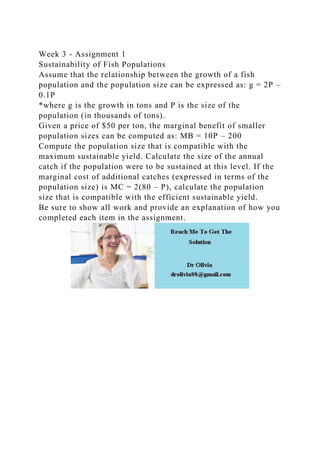Successfully reported this slideshow.

# Week 3 - Assignment 1Sustainability of Fish PopulationsAssume th.docx×

# Week 3 - Assignment 1Sustainability of Fish PopulationsAssume th.docx

Week 3 - Assignment 1
Sustainability of Fish Populations
Assume that the relationship between the growth of a fish population and the population size can be expressed as: g = 2P – 0.1P
*where g is the growth in tons and P is the size of the population (in thousands of tons).
Given a price of \$50 per ton, the marginal benefit of smaller population sizes can be computed as: MB = 10P – 200
Compute the population size that is compatible with the maximum sustainable yield. Calculate the size of the annual catch if the population were to be sustained at this level. If the marginal cost of additional catches (expressed in terms of the population size) is MC = 2(80 – P), calculate the population size that is compatible with the efficient sustainable yield.
Be sure to show all work and provide an explanation of how you completed each item in the assignment.
.

Week 3 - Assignment 1
Sustainability of Fish Populations
Assume that the relationship between the growth of a fish population and the population size can be expressed as: g = 2P – 0.1P
*where g is the growth in tons and P is the size of the population (in thousands of tons).
Given a price of \$50 per ton, the marginal benefit of smaller population sizes can be computed as: MB = 10P – 200
Compute the population size that is compatible with the maximum sustainable yield. Calculate the size of the annual catch if the population were to be sustained at this level. If the marginal cost of additional catches (expressed in terms of the population size) is MC = 2(80 – P), calculate the population size that is compatible with the efficient sustainable yield.
Be sure to show all work and provide an explanation of how you completed each item in the assignment.
.